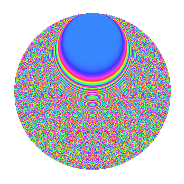# Properties

 Label 525.4.bkLevel $525$ Weight $4$ Character orbit 525.bk Rep. character $\chi_{525}(8,\cdot)$ Character field $\Q(\zeta_{20})$ Dimension $1440$ Sturm bound $320$

# Related objects

## Defining parameters

 Level: $$N$$ $$=$$ $$525 = 3 \cdot 5^{2} \cdot 7$$ Weight: $$k$$ $$=$$ $$4$$ Character orbit: $$[\chi]$$ $$=$$ 525.bk (of order $$20$$ and degree $$8$$) Character conductor: $$\operatorname{cond}(\chi)$$ $$=$$ $$75$$ Character field: $$\Q(\zeta_{20})$$ Sturm bound: $$320$$

## Dimensions

The following table gives the dimensions of various subspaces of $$M_{4}(525, [\chi])$$.

Total New Old
Modular forms 1952 1440 512
Cusp forms 1888 1440 448
Eisenstein series 64 0 64

## Trace form

 $$1440q - 8q^{3} + O(q^{10})$$ $$1440q - 8q^{3} - 144q^{10} + 128q^{12} + 144q^{13} + 16q^{15} + 5760q^{16} - 460q^{18} - 240q^{19} + 144q^{22} + 2376q^{25} + 592q^{27} + 580q^{30} + 56q^{33} - 5280q^{34} - 2088q^{37} - 2440q^{39} - 144q^{40} - 560q^{42} - 240q^{43} + 880q^{45} + 9012q^{48} - 240q^{52} + 7540q^{54} - 1200q^{55} + 1112q^{57} - 840q^{58} + 6308q^{60} - 4256q^{63} + 5760q^{64} + 1392q^{67} + 1008q^{70} - 18744q^{72} - 7536q^{73} - 5280q^{75} - 7900q^{78} - 4320q^{79} + 1280q^{81} - 18240q^{82} - 13224q^{85} + 8592q^{87} + 18672q^{88} + 5664q^{90} + 10848q^{93} + 38640q^{94} + 7872q^{97} + O(q^{100})$$

## Decomposition of $$S_{4}^{\mathrm{new}}(525, [\chi])$$ into newform subspaces

The newforms in this space have not yet been added to the LMFDB.

## Decomposition of $$S_{4}^{\mathrm{old}}(525, [\chi])$$ into lower level spaces

$$S_{4}^{\mathrm{old}}(525, [\chi]) \cong$$ $$S_{4}^{\mathrm{new}}(75, [\chi])$$$$^{\oplus 2}$$

## Hecke characteristic polynomials

There are no characteristic polynomials of Hecke operators in the database